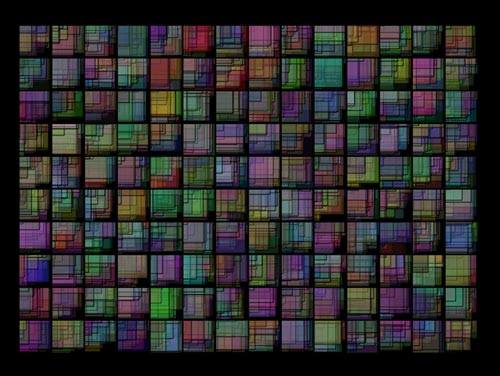Categories

# Processing: Square CityFor our latest installment of Processing Month, we’ve got a lot of squares, er, rectangles. They overlap. They are semi-transparent.

Another simple sketch, with randomness in it.

```/*
* 20110504.pde
*/

void setup() {
size(800, 600);
background(0);
}

float x = 50;
float y = 50;
int   c = 0;

void draw() {

fill(random(0,200),random(0,200),random(0,200), 70);
strokeWeight(0);
rect(x, y, random(1,50), random(1,50));

if (x > 650) {
x = 50;
y = y + 50;
}
else {
x = x + 50;
}

if (y > 500) {
y = 50;
if (c > 500) {
background(0);
c = 0;
}
c++;
}
}
```

It draws rectangles on top of rectangles on top of rectangles, and eventually clears the screen after enough iterations.

See any improvements you would make? Let me know…